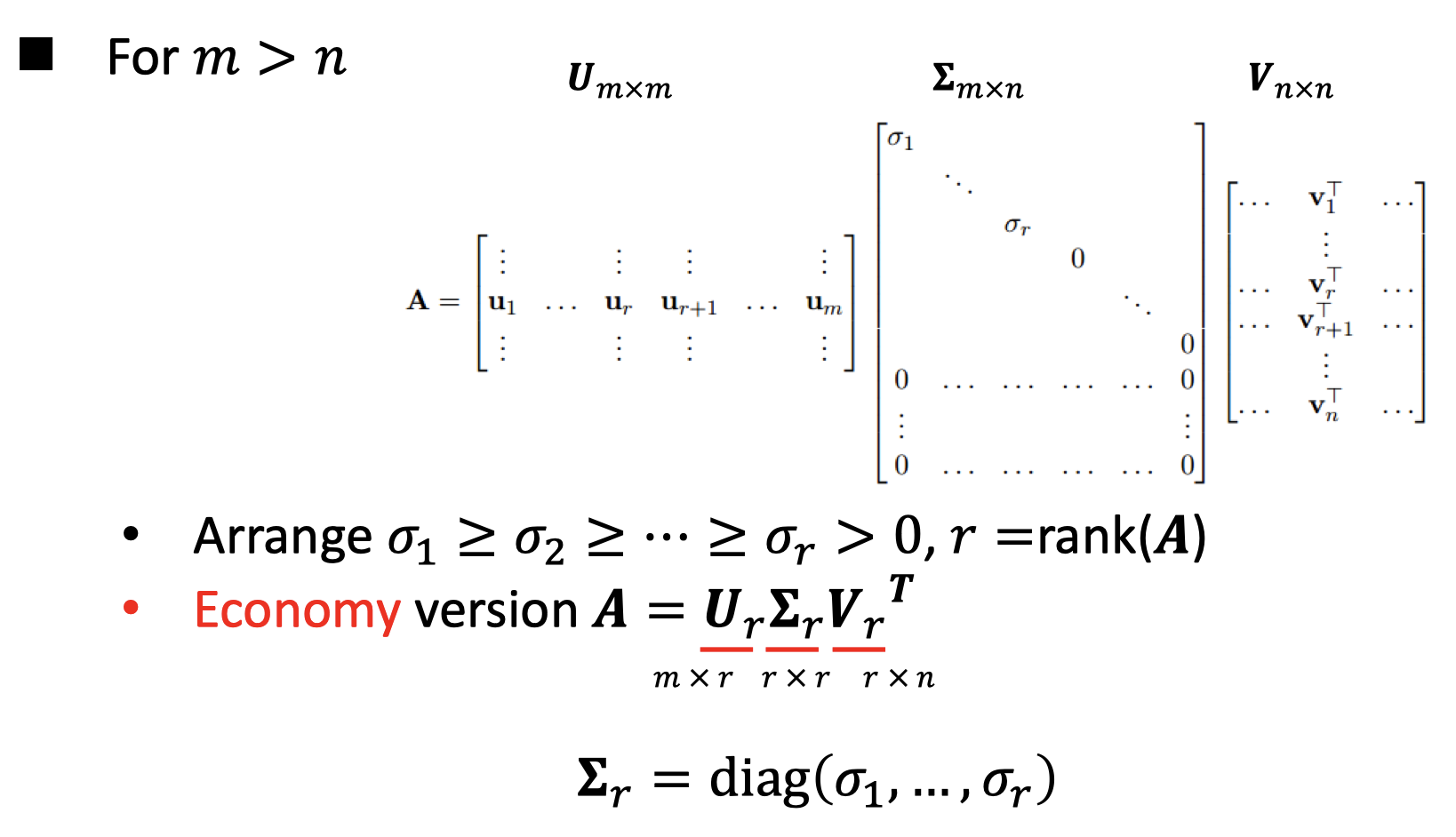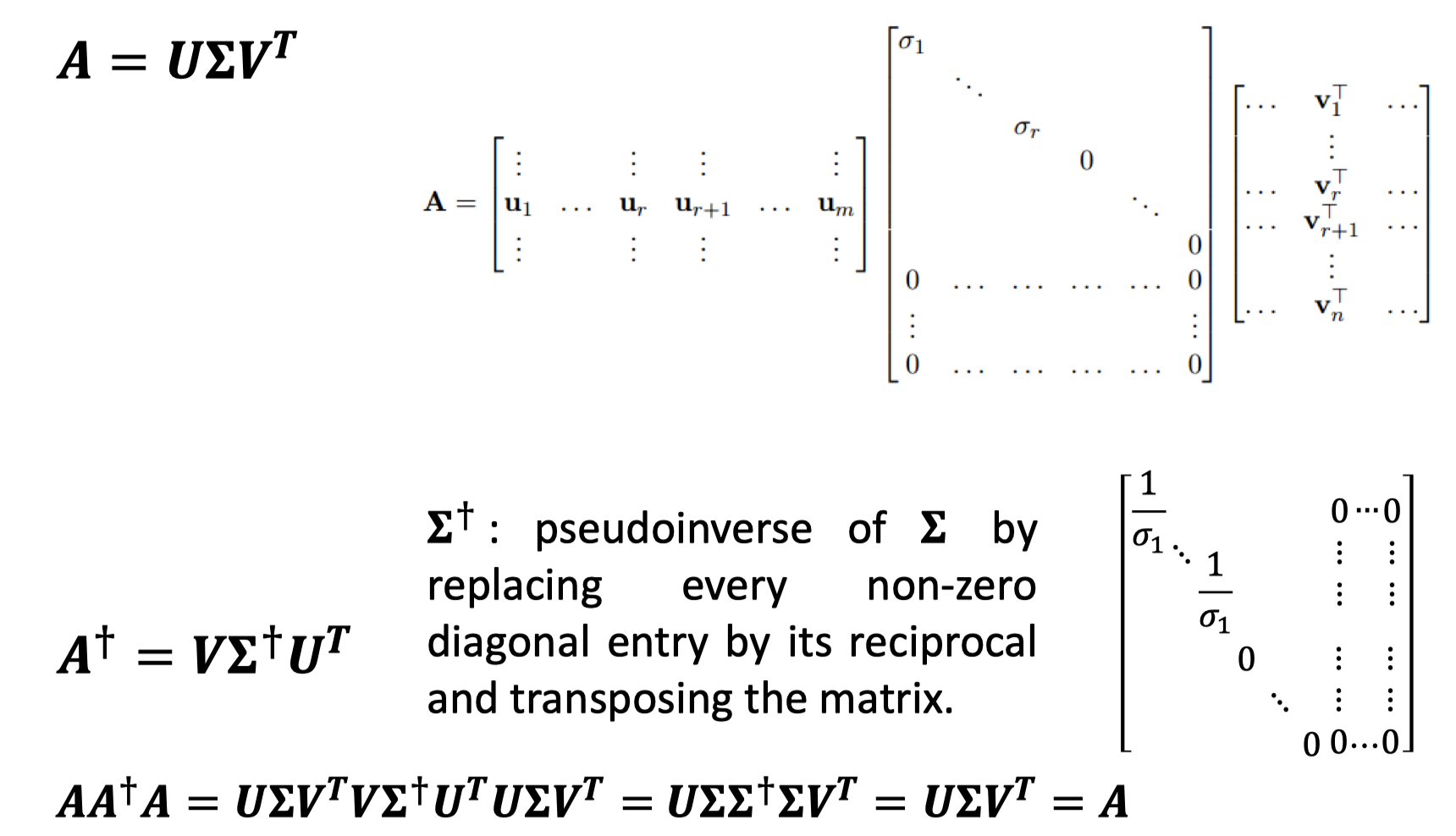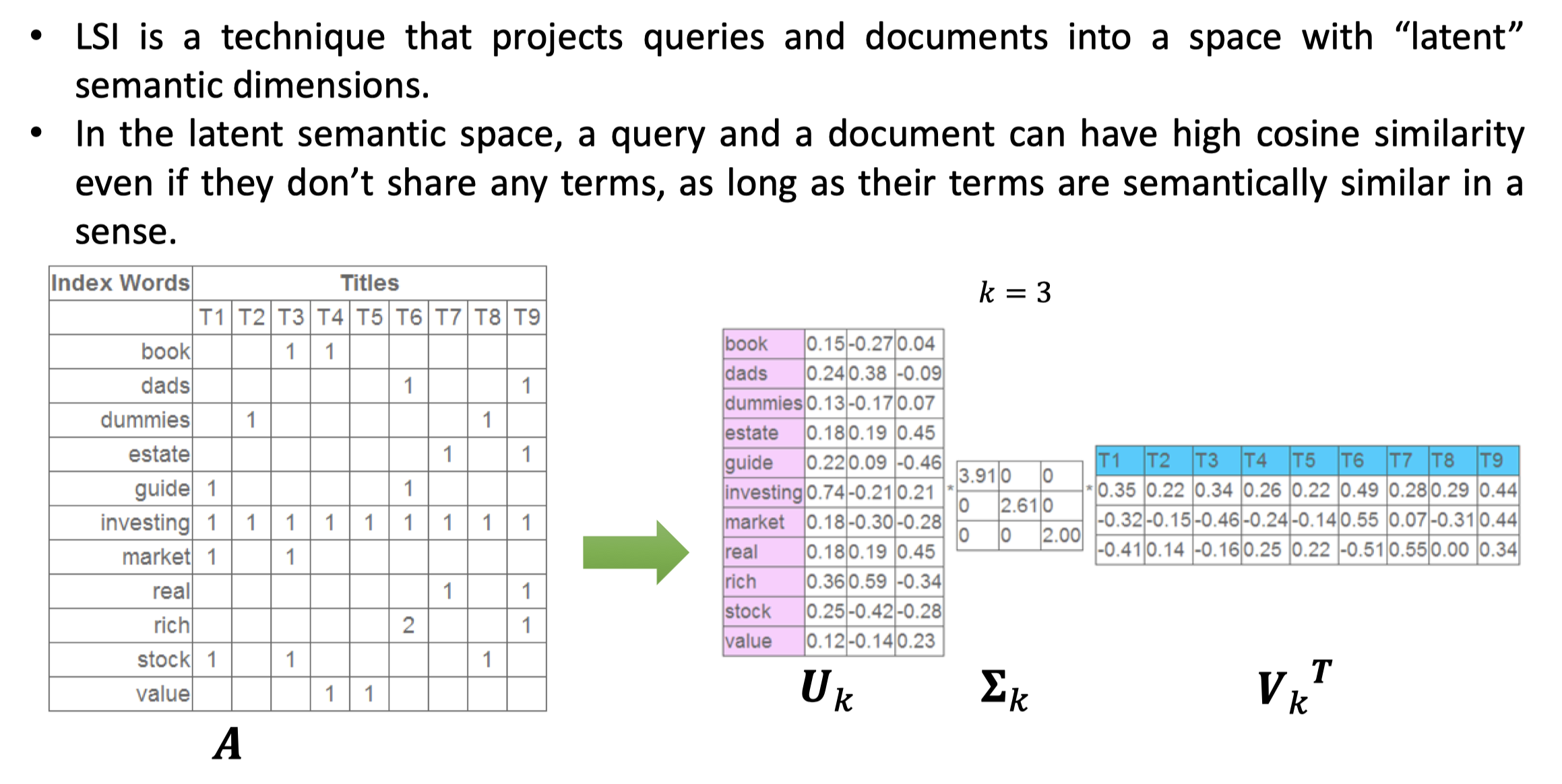• 实对称矩阵的特征值求法_实对称矩阵、相似、标准型、合同的逻辑网
千次阅读
2020-11-20 15:49:53

考虑充要条件==，

矩阵A、B相似==A、B特征值相同且A、B均可相似标准化(特征对角阵化)——1

矩阵A、B合同==A、B有相同正负惯性指数——2

矩阵A、B均以正交变换进行相似对角化，即A、B均与各自相似标准型合同==A、B与各自对应的二次型，均可化为系数为特征值的标准二次型（特征标准二次型）——3

上述一条，是因为：

f（x1、x2、x3）=xTAx。对f进行变换，即对x进行线性变化，即x=Cy。代入左式，得f（y1、y2、y3）=yTCTACy=yT（CTAC）y。由此可见，对二次型做变换，必是对其矩阵做合同变换；对二次型的矩阵做合同变换，必可转换为对二次型做变换。而对二次型矩阵仅仅做相似变换C-1BC=A，不能转换为对相应二次型做变换。因为yTC-1ACy无法通过x=Cy转变为xTCx 。

2+3，得：矩阵A、B均与各自相似标准型合同，且A、B的特征值的正负个数相同==矩阵A、B合同——4

矩阵是实对称矩阵，则必可同时相似、合同于它的相似标准型。所以实对称矩阵A、B有相同特征值==A、B相似；实对称矩阵A、B正负特征值个数相同==A、B合同

对于二次型，二次型的标准型不唯一，但特征标准型唯一，系数即为特征值。

二次型的特征标准型唯一，但它的正交矩阵不唯一。原二次型矩阵以特征向量为基础，选择不同的施密特正交化方式（beta1=α1，α1可从不正交的一组向量中任意选择，因此施密特正交化可得不同的正交向量组），即可得不同的正交矩阵。

二次型的标准型不唯一，但规范型必唯一，原因是标准型的系数可任意取，而规范型的系数只取1、-1、0。在向量、系数、二次型函数值三个相关量中，标准型有系数和向量两个自由度，减去相关性还有一个自由度，因此可以自由转换；而规范型的系数、二次型函数值都不自由，强迫第三个相关量，即向量也不自由，自由度为零，因此表达式唯一。

更多相关内容
• 计算许多3x3实对称矩阵的特征值。 计算是非迭代的，基于完全矢量化的 matlab 矩阵运算，并且支持 GPU 计算。 它可以快速有效地同时处理多个 3×3 矩阵。 此代码特别适合 3D 中的张量/黎曼微积分、体积张量图像的可视...matlab
• 2阶实对称矩阵特征值和特征向量的简单求解方法。因为2阶实对称矩阵的特殊性，可以直接使用初中的2阶方程 x = -b±sqrt(b*b -4*a*c) / 2*a进行求解。这个方法在求解平面点的hessian矩阵很有用处。
• 本文主要针对线性代数中的正定矩阵实对称矩阵矩阵特征值分解以及矩阵 SVD 分解进行总结。 如果你对这篇文章可感兴趣，可以点击「【访客必读 - 指引页】一文囊括主页内所有高质量博客」，查看完整博客分类与对应...

## 前言

本文主要针对线性代数中的正定矩阵、实对称矩阵、矩阵特征值分解以及矩阵 SVD 分解进行总结。

如果你对这篇文章可感兴趣，可以点击「【访客必读 - 指引页】一文囊括主页内所有高质量博客」，查看完整博客分类与对应链接。

## 正定矩阵

### 1. 概念

首先正定矩阵是定义在对称矩阵的基础上，其次对于任意非零向量 x \textbf{x} ，若 x T A x > 0 \textbf{x}^T\textbf{\textit{A}}\textbf{x}>0 恒成立，则矩阵 A \textbf{\textit{A}} 为正定矩阵；若 x T A x ≥ 0 \textbf{x}^T\textbf{\textit{A}}\textbf{x}\geq 0 恒成立，则矩阵 A \textbf{\textit{A}} 为半正定矩阵。

### 2. 物理意义

任意非零向量 x \textbf{x} 经过矩阵 A A 线性变换后，与原先向量的夹角 ≤ 90 \leq 90 度。

### 3. 其他充要条件

• 充要条件1： 矩阵 A \textbf{\textit{A}} 的全部特征值都是正数
• 推论： A \textbf{\textit{A}} 正定，则 ∣ A ∣ > 0 |\textbf{\textit{A}}|>0 ，即 A \textbf{\textit{A}} 可逆（有时会根据矩阵正定来判断是否可逆）
• 推论： A \textbf{\textit{A}} 正定，则 A \textbf{\textit{A}} 与单位阵合同，即存在可逆阵 C \textbf{\textit{C}} ，使得 C T AC = E \textbf{\textit{C}}^T\textbf{\textit{A}}\textbf{\textit{C}}=\textbf{\textit{E}} 成立
• 充要条件2： 矩阵 A \textbf{\textit{A}} 的各阶顺序主子式都是正数，即 Δ i > 0 \Delta_i>0
• 其中 Δ i \Delta_i 表示矩阵 A \textbf{\textit{A}} i i 行与前 i i 列组成的子矩阵的行列式的值
• 推论： ∣ A ∣ > 0 |A|>0 A A 一定可逆

## 实对称矩阵

### 1. 概念

矩阵为方阵，其中元素均为实数，且 A = A T \textbf{\textit{A}}=\textbf{\textit{A}}^T

### 2. 性质

• 性质1： 实对称矩阵的特征值都是实数。
• 假设 λ \lambda x \textbf{x} 分别为矩阵 A \textbf{\textit{A}} 的特征值、特征向量，即 A x = λ x \textbf{\textit{A}}\textbf{x}=\lambda \textbf{x}
• 等式两边取共轭，即 a + b i ‾ = a − b i \overline{a+bi}=a-bi A ‾ x ‾ = λ ‾ x ‾ \overline{\textbf{\textit{A}}}\overline{\textbf{x}}=\overline{\lambda} \overline{\textbf{x}} A \textbf{\textit{A}} 是实对称矩阵，因此 A = A T = A ‾ \textbf{\textit{A}}=\textbf{\textit{A}}^T=\overline{\textbf{\textit{A}}} ，即 A x ‾ = λ ‾ x ‾ \textbf{\textit{A}}\overline{\textbf{x}}=\overline{\lambda} \overline{\textbf{x}}
• 等式两边取转置，则 x T A = λ x T \textbf{x}^T\textbf{\textit{A}}=\lambda \textbf{x}^T
• x T A x ‾ = λ ‾ x T x ‾ = λ x T x ‾ \textbf{x}^T\textbf{\textit{A}}\overline{x}=\overline{\lambda}\textbf{x}^T\overline{\textbf{x}}=\lambda \textbf{x}^T\overline{\textbf{x}}
• ( λ − λ ‾ ) ∥ x ∥ 2 2 = 0 (\lambda-\overline{\lambda})\left\|\textbf{x}\right\|_2^2=0 ，由于 ∥ x ∥ 2 2 > 0 \left\|\textbf{x}\right\|_2^2>0 ，因此 λ = λ ‾ \lambda=\overline{\lambda} λ \lambda 为实数
• 性质2： 实对称矩阵不同特征值所对应的特征向量必定正交。
• 假设 A x 1 = λ 1 x 1 \textbf{\textit{A}}\textbf{x}_1=\lambda_1 \textbf{x}_1 A x 2 = λ 2 x 2 \textbf{\textit{A}}\textbf{x}_2=\lambda_2 \textbf{x}_2 成立
• x 1 T A = λ 1 x 1 T \textbf{x}_1^T\textbf{\textit{A}}=\lambda_1 \textbf{x}_1^T
• x 1 T A x 2 = λ 1 x 1 T x 2 = λ 2 x 1 T x 2 \textbf{x}_1^T\textbf{\textit{A}}\textbf{x}_2=\lambda_1 \textbf{x}_1^T\textbf{x}_2=\lambda_2\textbf{x}_1^T\textbf{x}_2
• ( λ 1 − λ 2 ) x 1 T x 2 = 0 (\lambda_1-\lambda_2)\textbf{x}_1^T\textbf{x}_2=0 ，因此 x 1 \textbf{x}_1 x 2 \textbf{x}_2 正交
• 性质3： 实对称矩阵相同特征值所对应的特征向量必定线性无关。
• 证明较繁琐，不详细展开
• 线性无关的向量可以通过施密特正交化转为正交向量
• 对于线性无关向量组 x 1 , x 2 , . . . , x n \textbf{x}_1,\textbf{x}_2,...,\textbf{x}_n ，转为正交向量组 y 1 , y 2 , . . . , y n \textbf{y}_1,\textbf{y}_2,...,\textbf{y}_n
• y 1 = x 1 \textbf{y}_1=\textbf{x}_1
• y i = x i − ∑ j = 1 i − 1 x i T y j y j T y j y j \textbf{y}_i=\textbf{x}_i-\sum\limits_{j=1}^{i-1}\displaystyle\frac{\textbf{x}_i^T\textbf{y}_j}{\textbf{y}_j^T\textbf{y}_j}\textbf{y}_j
• 由于新的正交向量都是原来线性无关向量的线性组合，而原先的线性无关向量对应的特征值均相同，因此新的正交向量也均为该相同特征值对应的特征向量
• 性质4： 任何一个实对称矩阵，都可以正交对角化。
• 正交对角化，即存在一个正交矩阵 Q ( Q T = Q − 1 ) \textbf{\textit{Q}}(\textbf{\textit{Q}}^T=\textbf{\textit{Q}}^{-1}) 使得 Q T AQ = D \textbf{\textit{Q}}^T\textbf{\textit{A}}\textbf{\textit{Q}}=\textbf{\textit{D}} ，其中 D \textbf{\textit{D}} 是一个对角矩阵
• 实对称矩阵，一定有 n n 个解，因为实对称矩阵特征值都是实数，因此一共有 n n 个实特征值（包括重特征值）—— 性质 1 1
• 不同特征值对应的特征向量正交，相同特征值也一定存在对应的正交向量 —— 性质 2 , 3 2,3
• 实对称矩阵，一定有 n n 个正交特征向量，因此可以特征值分解，即该性质成立
• 性质5： 实对称矩阵的非零特征值个数等于矩阵的秩
• 矩阵 A \textbf{\textit{A}} 相似于对角矩阵， P − 1 AP = D \textbf{\textit{P}}^{-1}\textbf{\textit{A}}\textbf{\textit{P}}=\textbf{\textit{D}}
• 对角矩阵 D \textbf{\textit{D}} 的秩 = 矩阵 A \textbf{\textit{A}} 的秩 = D \textbf{\textit{D}} 非零特征值个数
• 矩阵 A \textbf{\textit{A}} 与 矩阵 D \textbf{\textit{D}} 相似，则特征值相同
• 性质6：实对称矩阵不一定可逆，但若可逆，则一定是实对称矩阵
• 0 矩阵对称不可逆
• ( A − 1 ) T = ( A T ) − 1 = A − 1 (A^{-1})^T=(A^T)^{-1}=A^{-1}

## 矩阵特征值分解

### 1. 概念

n ∗ n n*n 的方阵 A \textbf{\textit{A}} ，由 A x = λ x \textbf{\textit{A}}\textbf{x}=\lambda \textbf{x} 可以得到 AV = V Λ \textbf{\textit{A}}\textbf{\textit{V}}=\textbf{\textit{V}}\Lambda

• 如果方阵 A \textbf{\textit{A}} n n 个线性无关的特征向量，则 V \textbf{\textit{V}} 可逆
• A = V Λ V − 1 \textbf{\textit{A}}=\textbf{\textit{V}}\Lambda\textbf{\textit{V}}^{-1}
• 其中矩阵 V \textbf{\textit{V}} 的列为方阵 A \textbf{\textit{A}} 的特征向量， Λ = d i a g ( λ 1 , λ 2 , . . . , λ n ) , λ i ≥ λ i + 1 \Lambda=diag(\lambda_1,\lambda_2,...,\lambda_n),\lambda_i\geq \lambda_{i+1}

## 矩阵 SVD 分解

### 1. 概念

任意一个矩阵 A \textbf{\textit{A}} 都可以分解为 A = U Σ V T \textbf{\textit{A}}=\textbf{\textit{U}}\Sigma\textbf{\textit{V}}^T ，其中 U , V \textbf{\textit{U}},\textbf{\textit{V}} 均为正交单位矩阵， Σ \Sigma 为对角矩阵。

### 2. 证明

• A T A = ( U Σ V T ) T U Σ V T = V Σ 2 V T \textbf{\textit{A}}^T\textbf{\textit{A}}=(\textbf{\textit{U}}\Sigma\textbf{\textit{V}}^T)^T\textbf{\textit{U}}\Sigma\textbf{\textit{V}}^T=\textbf{\textit{V}}\Sigma^2\textbf{\textit{V}}^T ，由于 A T A \textbf{\textit{A}}^T\textbf{\textit{A}} 为实对称矩阵，因此 V \textbf{\textit{V}} 为矩阵 A T A \textbf{\textit{A}}^T\textbf{\textit{A}} 对应特征向量组成的正交单位阵。
• A A T = U Σ V T ( U Σ V T ) T = U Σ 2 U T \textbf{\textit{A}}\textbf{\textit{A}}^T=\textbf{\textit{U}}\Sigma\textbf{\textit{V}}^T(\textbf{\textit{U}}\Sigma\textbf{\textit{V}}^T)^T=\textbf{\textit{U}}\Sigma^2\textbf{\textit{U}}^T ，由于 A A T \textbf{\textit{A}}\textbf{\textit{A}}^T 为实对称矩阵，因此 U \textbf{\textit{U}} 矩阵 A A T \textbf{\textit{A}}\textbf{\textit{A}}^T 对应特征向量组成的正交单位阵。
• AV = U Σ \textbf{\textit{A}}\textbf{\textit{V}}=\textbf{\textit{U}}\Sigma ，其中 Σ \Sigma 为对角阵，因此 A v i = σ i u i \textbf{\textit{A}}\textbf{v}_i=\sigma_i\textbf{u}_i ，由此可以得到对角矩阵 Σ \Sigma ，其中 σ i \sigma_i 就是奇异值。
• A m ∗ n = U m ∗ m Σ m ∗ n V n ∗ n T \textbf{\textit{A}}_{m*n}=\textbf{\textit{U}}_{m*m}\Sigma_{m*n}\textbf{\textit{V}}_{n*n}^T

### 3. 几何角度

矩阵 U , V U,V 仅负责旋转， Σ \Sigma 负责放缩，具体示意图如下：### 4. SVD 压缩

如下所示，仅选取前 r r 个不为零的奇异值，可以实现无损压缩。注意非零奇异值的个数等于矩阵 A A 的秩。### 5. 计算伪逆### 6. Eckart-Young Theorem

如果矩阵 B \mathbf{B} 的秩为 k k ，则 ∣ ∣ A − B ∣ ∣ ≥ ∣ ∣ A − A k ∣ ∣ ||A-B||\geq||A-A_k|| 对如下三个矩阵范数成立：

• ∣ ∣ A ∣ ∣ 2 = σ 1 ||A||_2=\sigma_1 ，即最大的奇异值
• ∣ ∣ A ∣ ∣ N u c l e a r = ∑ i = 1 r σ i ||A||_{Nuclear}=\sum\limits_{i=1}^r\sigma_i
• Frobenius norm = ∣ ∣ A ∣ ∣ 2 , 1 = ∣ ∣ A ∣ ∣ F = ( t r ( A T A ) ) 1 / 2 = ( ∑ i = 1 m ∑ j = 1 n a i j 2 ) 1 / 2 =||A||_{2,1}=||A||_F=(tr(A^TA))^{1/2}=(\sum\limits_{i=1}^m\sum\limits_{j=1}^na_{ij}^2)^{1/2}

其中 A \mathbf{A} A k \mathbf{A_k} 定义如下：
A = U Σ V T = ∑ i = 1 r σ i u i v i T A k = U k Σ k V k T = ∑ i = 1 k σ i u i v i T \begin{aligned} & \mathbf{A}=\mathbf{U}\Sigma\mathbf{V}^T=\sum\limits_{i=1}^r \sigma_i\mathbf{u}_i\mathbf{v}_i^T\\ & \mathbf{A}_k=\mathbf{U}_k\Sigma_k\mathbf{V}_k^T=\sum\limits_{i=1}^k \sigma_i\mathbf{u}_i\mathbf{v}_i^T \end{aligned}

需要注意，矩阵乘上一个正交矩阵，其奇异值不会发生变化，即上述涉及的矩阵范数不会改变。

### 7. LSI

计算不同 q u e r y query 之间的相似程度，常用于推荐系统。更多 SVD 的应用：

展开全文• 对于任意的nnn阶实对称矩阵AAA，存在正交矩阵QQQ，使得 Q−1AQ=QTAQ=diag(λ1,…,λn)Q^{-1}AQ=Q^T AQ=diag(\lambda_1,\dots,\lambda_n)Q−1AQ=QTAQ=diag(λ1​,…,λn​) 其中λ1,…,λn\lambda_1,\dots,\lambda_n...

对于任意的 n n 阶实对称矩阵 A A ，存在正交矩阵 Q Q ，使得
Q − 1 A Q = Q T A Q = d i a g ( λ 1 , … , λ n ) Q^{-1}AQ=Q^T AQ=diag(\lambda_1,\dots,\lambda_n)
其中 λ 1 , … , λ n \lambda_1,\dots,\lambda_n A A 的特征值

证明：
n = 1 n=1
I − 1 A I = a 11 I^{-1}AI=a_{11}
成立
假设当 n = k − 1 n=k-1 时成立
不妨设 A α 1 = λ 1 α 1 ( ∥ α 1 ∥ 2 = 1 ) A\alpha_1=\lambda_1 \alpha_1(\Vert \alpha_1 \Vert_2=1)
由施密特正交化，存在 n − 1 n-1 个单位向量 α 2 , α 3 , … , α n \alpha_2,\alpha_3,\dots,\alpha_n (其中 α 2 , α 3 , … , α n \alpha_2,\alpha_3,\dots,\alpha_n 不一定是特征值)
使得 α 1 , α 2 , … , α n \alpha_1,\alpha_2,\dots,\alpha_n 两两正交
Q 1 = ( α 1 , α 2 , … , α n ) Q_1=(\alpha_1,\alpha_2,\dots,\alpha_n)
Q 1 T A Q 1 = ( α 1 T A α 1 α 1 T A α 2 α 1 T A α 3 ⋯ α 1 T A α n α 2 T A α 1 α 2 T A α 2 α 2 T A α 3 ⋯ α 2 T A α n ⋯ ⋯ ⋯ ⋯ ⋯ α n T A α 1 α n T A α 2 α n T A α 3 ⋯ α n T A α n ) = ( λ 1 α 1 T α 1 λ 1 α 1 T α 2 λ 1 α 1 T α 3 ⋯ λ 1 α 1 T α n λ 1 α 2 T α 1 α 2 T A α 2 α 2 T A α 3 ⋯ α 2 T A α n ⋯ ⋯ ⋯ ⋯ ⋯ λ 1 α n T α 1 α n T A α 2 α n T A α 3 ⋯ α n T A α n ) = ( λ 1 0 0 ⋯ 0 0 α 2 T A α 2 α 2 T A α 3 ⋯ α 2 T A α n ⋯ ⋯ ⋯ ⋯ ⋯ 0 α n T A α 2 α n T A α 3 ⋯ α n T A α n ) = ( λ 1 0 0 S ) \begin{aligned} Q_1^TAQ_1&=\begin{pmatrix} \alpha_1^TA\alpha_1&\alpha_1^TA\alpha_2&\alpha_1^TA\alpha_3&\cdots &\alpha_1^TA\alpha_n\\ \alpha_2^TA\alpha_1&\alpha_2^TA\alpha_2&\alpha_2^TA\alpha_3&\cdots &\alpha_2^TA\alpha_n\\ \cdots&\cdots&\cdots&\cdots&\cdots\\ \alpha_n^TA\alpha_1&\alpha_n^TA\alpha_2&\alpha_n^TA\alpha_3&\cdots &\alpha_n^TA\alpha_n\\ \end{pmatrix}\\ &=\begin{pmatrix} \lambda_1\alpha_1^T\alpha_1&\lambda_1\alpha_1^T\alpha_2&\lambda_1\alpha_1^T\alpha_3&\cdots &\lambda_1\alpha_1^T\alpha_n\\ \lambda_1\alpha_2^T\alpha_1&\alpha_2^TA\alpha_2&\alpha_2^TA\alpha_3&\cdots &\alpha_2^TA\alpha_n\\ \cdots&\cdots&\cdots&\cdots&\cdots\\ \lambda_1\alpha_n^T\alpha_1&\alpha_n^TA\alpha_2&\alpha_n^TA\alpha_3&\cdots &\alpha_n^TA\alpha_n\\ \end{pmatrix}\\ &=\begin{pmatrix} \lambda_1&0&0&\cdots &0\\ 0&\alpha_2^TA\alpha_2&\alpha_2^TA\alpha_3&\cdots &\alpha_2^TA\alpha_n\\ \cdots&\cdots&\cdots&\cdots&\cdots\\ 0&\alpha_n^TA\alpha_2&\alpha_n^TA\alpha_3&\cdots &\alpha_n^TA\alpha_n\\ \end{pmatrix}\\ &=\begin{pmatrix} \lambda_1&0\\ 0&S\\ \end{pmatrix} \end{aligned}
S S 是一个 n − 1 n-1 阶实对称矩阵
由假设
存在正交矩阵 Q Q ,使得
Q T S Q = d i a g ( λ 2 , λ 3 , … , λ n ) Q^T SQ=diag(\lambda_2,\lambda_3,\dots,\lambda_n)
Q 2 = ( 1 0 0 Q ) Q_2=\begin{pmatrix} 1&0\\ 0&Q \end{pmatrix}
显然 Q 2 Q_2 n n 阶正交矩阵
Q 2 T Q 1 T A Q 1 Q 2 = ( Q 1 Q 2 ) T A ( Q 1 Q 2 ) = d i a g ( λ 1 , … , λ n ) Q_2^T Q_1^TAQ_1 Q_2=(Q_1 Q_2)^TA(Q_1 Q_2)=diag(\lambda_1,\dots,\lambda_n)
显然 Q 1 Q 2 Q_1 Q_2 是正交矩阵
由数学归纳法，结论成立

# 推论

Q T A Q = d i a g ( λ 1 , … , λ n ) Q^TAQ=diag(\lambda_1,\dots,\lambda_n)
A Q = Q d i a g ( λ 1 , … , λ n ) AQ=Qdiag(\lambda_1,\dots,\lambda_n)
( A x 1 , … , A x n ) = ( λ 1 x 1 , … , λ n x n ) (Ax_1,\dots,Ax_n)=(\lambda_1 x_1,\dots,\lambda_n x_n)
A x i = λ i x i ( i = 1 , 2 , … , n ) Ax_i=\lambda_i x_i(i=1,2,\dots,n)
显然 Q Q A A 的特征向量经过施密特正交化和单位化后得到的正交矩阵

接着证明这个对角矩阵取遍了所有 A A 的特征值，且 k k 重特征值取了 k k

等价于证明，实对称矩阵 k k 重特征值恰好有 k k 个线性无关的特征向量

由几何重复度小于等于代数重复度（by 矩阵论)
( A − λ i ) x = 0 (A-\lambda_i)x=0 的基础解系线性无关向量的个数，不会超过 λ i \lambda_i 的重数
假设 λ i \lambda_i 的线性无关特征向量小于 k k ，则必然有一个特征值对应的线性无关特征向量多了一个，矛盾

展开全文• 一、正交向量组与正交矩阵 正交向量组的定义，是一组非零向量，且两两正交，那么这组向量，则成为正交向量组。 两个向量正交的意思是，两个向量的内积为0，什么是两个向量的内积，就是向量内对应元素的积的和。 ...

一、正交向量组与正交矩阵

正交向量组的定义是一组非零向量，且两两正交，那么这组向量，则成为正交向量组。

两个向量正交的意思是，两个向量的内积为0，什么是两个向量的内积，就是向量内对应元素的积的和。

，两个向量的内积为，

正交单位向量组的定义

一组非零向量是正交向量组，在此基础上，每个向量还是单位向量，则称为正交单位向量组。

什么是单位向量，即该向量的模为1，例如的模为，

性质：

1、正交向量组必然线性无关

证明：设

如果我们能证这个式子里的k1，k2....kn只能为0的话，那么这几个向量必然是线性无关的

将这个式子左乘以，这样结果就是向量之间的内积了

又因为这个向量组是正交向量组，两两之间的内积都为0

因为是非零向量，这个就是a1的模，不为零，所以k1只能为0，同理，将其他向量的转置左乘这个式子，可以分别求出对应的k为0

所以正交向量组里的向量都是线性无关的。

正交矩阵的定义

若A为n阶方阵，且，则称A为一个正交矩阵

意味着，因为矩阵逆的定义是

正交矩阵的性质

1、每个行（列）向量都是单位向量

2、任意2个行（列）向量相互正交

3、正交矩阵的行列式为

证明：

4、如果A是正交矩阵，则A可逆，且A的逆与A的伴随都是正交矩阵

证明：A当然可逆了，A的转置就是A的逆了，A转置必然存在

A的转置与A互为正交矩阵，都满足正交矩阵的定义

5、如果A、B都是正交矩阵，那么AB与BA也是正交矩阵

二、实对称矩阵的特征值与特征向量的性质

1、什么是实对称阵？

实对称阵的定义：实对称阵是个方阵，特征值全为实数（普通方阵的特征值有可能为复数），并且是个对称阵，即，实对称阵不同特征值的特征向量之间不但无关，而且相互正交，并且必然可以相似对角化

我们称A可以相似对角化

当A是实对称阵的时候，上述等式成立，并且P为一个正交单位矩阵的时候，我们称之为实对称阵的正交相似对角化

这个式子我们知道，P是由A的特征向量组成的，是A的特征值

那么如何求得P？

因为实对称阵性质的不同特征值下的特征向量必然是线性无关且相互正交的，那么当A的特征值各不相同的情况下，A的特征向量必然相互正交

但是当A有多重特征值的时候，比如有2个相同的特征值，那么对该特征值对应的2个特征向量进行正交化就行。

施密特正交法：将多重特征值下的特征向量正交化

例如将正交化

，括号的意思是求内积

就是处理过后的正交向量

最后，再对所有的特征向量进行单位化即可（因为我们知道，k倍的特征向量还是特征向量）

展开全文• 考虑以下问题:问题Ⅰ:给定矩阵X∈Rn×m,D∈SRm×m,求(A,B)∈SRn×n,使满足AX =BXD,其中SRn×n为n阶实对称矩阵的集合.问题Ⅱ:给定A^∈Rn×n,B^ )∈Rn×n,求(A^,B^ )∈SAB,使得‖(A^,B^ ) - ( A^,B^ )‖F= inf ∨(A^,B...
• 实对称矩阵：如果有n阶矩阵A，其矩阵的元素都为实数，且矩阵A的转置等于其本身（aij=aji）(i,j为元素的脚标），则称A为实对称矩阵。 两个概念的不同之处在于：实对称矩阵里的元素全是实数，而对称矩阵只说明A^T=A，...
• 矩阵与对角形相似(P−1AP=ΛP^{-1}AP=\LambdaP−1AP=Λ)的条件 定理1 AAA 相似于对角形 Λ\LambdaΛ 的充要条件是 AAA 有 nnn 个线性无关的特征向量。 推论 ...所有的实对称矩阵都能对角化！！！ 向量
• 对称矩阵对称矩阵（Symmetric Matrix）是指元素以主对角线为对称轴对应相等的矩阵，例如： 可以看到，对称矩阵的转置等于其自身，即： 对角矩阵对角矩阵（Diagonal Matrix）是指除主对角线之外其他元素都为0的矩阵，...
• 本章是特征值与特征向量知识的延续，根据谱定理可知实对称矩阵可以正交对角化，对角为其特征值，正交矩阵为其两两正交的单位特征向量。除此之外，还介绍了二次型，标准形，规范形的知识。二次型的化简问题是本章的...
• ## 实对称矩阵一定可以对角化

万次阅读 多人点赞 2020-06-28 11:11:44
实对称矩阵一定可以对角化. 最近看共轭梯度下降的时候看到有人的推导里面用到了这个命题. 虽然以前学过, 但是学得很渣, 所以没有自己想过这个命题怎么样成立的. 现在将这些证明过程梳理一下. 实对称矩阵含有n个实根 ...
• 矩阵的特征值与特征向量的计算的matlab实现，幂法、反幂法和位移反幂法、雅可比(Jacobi)方法、豪斯霍尔德(Householder)方法、实对称矩阵的三对角化、QR方法、求根位移QR方法计算实对称矩阵 的特征值、广义特征值问题...
• 先上一道题，来自xqh博客每周一题设 为 阶实反对称矩阵， 为 阶实对称矩阵，证明： 均为非奇异（亦即： ）这道题有两种考虑方式一是考虑证明直接矩阵满秩，二是转化为求解特征值（即 证明 不是 的特征值）下面按两...
• 但是还原是不唯一的：二次型和一个实对称矩阵是可以对应的 简单的例子如下： 实对称矩阵 任何一个二次型，都可以写成 一横 一方 一竖 的形式： 就是 未知数 实对称矩阵 未知数： 现在在假设X=CY，其中C可逆 引入...
• 设AAA为实对称矩阵 A=A‾=A‾TA=\overline{A}=\overline{A}^TA=A=AT x‾TAx=x‾Tλx=λxTx\overline{x}^T Ax=\overline{x}^T\lambda x=\lambda x^T xxTAx=xTλx=λxTx x‾TA‾Tx=(A‾x‾)Tx=Ax‾Tx=λx‾Tx=λ‾x‾...
• ## 实对称矩阵

万次阅读 2018-10-18 19:14:30
实对称矩阵的定义，如果n阶矩阵A满足 则称A为实对称矩阵。 性质1：实对称矩阵的特征值都是实数 性质2：实对称属于不同特征值的特征向量正交 性质3：若是实对称矩阵A的k重特征值，则与对应的有k个线性无关的...
• ## 实对称矩阵性质

千次阅读 2021-06-21 11:02:27
n阶实对称阵必可以对角化, 且相似的对角阵的元素即是原矩阵的特征值; 若λk\lambda_{k}λk​具有k重特征值, 必有k个线性无关的特征向量, 或者说必有秩r(λ0E−A)=n−kr(\lambda_{0}E-A)=n-kr(λ0​E−A)=n−k. ...
•深度学习
• 1980年,Bellman,R.在文〔1〕中证明了下面...本文得到了与(1)、(2)类似的不等式tr((AB)~m)≤{tr(A~(2m))tr(B~(2m))}~(1/2) (3) 2tr((AB)~m)≤tr(A~(2m))+tr(B~(2m)) (4) 其中A、B是同阶实对称矩阵,m=2~k(k为非负整数)。
• 对于一个实对称矩阵不仅可以通过一个可逆矩阵相似对角化，还可以通过一个正交矩阵来相似对角化。实对称矩阵的不同特征值所对应的特征向量正交，而且实对称矩阵的特征值全为实数。在考研中，我们一定要重点掌握会求一...
• 《第四节 实对称矩阵的对角化》由会员分享，可在线阅读，更多相关《第四节 实对称矩阵的对角化(9页珍藏版)》请在人人文库网上搜索。1、一、实对称矩阵的性质,1,2,1,2,1,2,1,2,6.4.1,A,引理,设,是实对称矩阵,的两个...
• 对于实对称矩阵AAA，有J=P−1APJ=P^{-1}APJ=P−1AP,JJJ为AAA的若当标准型。 而 JT=PTAP−T=PTPP−1APP−1(P−1)T=(PTP)J(PTP)−1J^{T}=P^{T}AP^{-T}=P^TPP^{-1}APP^{-1}(P^{-1})^T=(P^TP)J(P^TP)^{-1}JT=PTAP−T=...
• 在线性代数中，有一些特殊的矩阵具有易于分析和操作的特性。它们的特征向量可能具有特定的特征值或特殊关系。还有一些方法可以将一个矩阵分解成这些“更简单”的矩阵。操作复杂性的降低提高了可伸缩性。然而，即使...
• 2.矩阵A的所有特征值的积等于A的行列式。3.关于A的矩阵多项式f(A)的特征值为f(μ)。4.若A可逆，则A−1的特征值为1/μ。5.若A与B相似，则A与B有相同特征多项式，即A与B特征值相同。6.属于A的不同特征值的特征向量线性...
• ## 实对称矩阵及其几大性质

万次阅读 多人点赞 2018-08-19 16:27:01
做机器学习的过程中，难免会与矩阵打交道，而实对称矩阵更是其中常用的矩阵之一。所以，下面将介绍一下什么是实对称矩阵，并介绍一下它的几个性质（这也是很多笔试题中常考的点） 定义：如果有n阶矩阵A，其矩阵的...
• n阶矩阵A可正交对角化的充分条件是A是实对称矩阵，即若A是实对称矩阵则A必可正交对角化。 首先，有以下定理： 若的特征值为，且，则存在正交矩阵Q，使A相似于如下三角矩阵： 证明如下（数学归纳法）： 设n*n阶...正交对角化
• 线性代数之 实对称矩阵，正交对角化，二次型与正定矩阵前言实对称矩阵正交对角化二次型正定矩阵实对称矩阵的正定判断条件后记 前言 终于快到矩阵分解了。在矩阵分解前，最后一个内容是实对称矩阵，二次型和正定矩阵...机器学习...